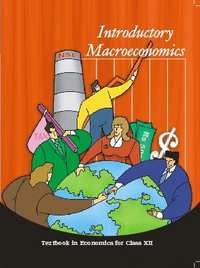Share

# NCERT solutions for Class 12 Economics chapter 6 - Open Economy Macroeconomics

#### NCERT Class 12 Economics - Introductory Macroeconomics## Chapter 6: Open Economy Macroeconomics

Exercise

#### NCERT solutions for Class 12 Economics Chapter 6 Exercise [Page 101]

Exercise | Q 1 | Page 101

Differentiate between balance of trade and current account balance.

Exercise | Q 2 | Page 101

What are official reserve transactions? Explain their importance in the balance of payments.

Exercise | Q 3 | Page 101

Distinguish between the nominal exchange rate and the real exchange rate. If you were to decide whether to buy domestic goods or foreign goods, which rate would be more relevant? Explain.

Exercise | Q 4 | Page 101

Suppose it takes 1.25 yen to buy a rupee, and the price level in Japan is 3 and the price level in India is 1.2. Calculate the real exchange rate between India and Japan (the price of Japanese goods in terms of Indian goods).

Exercise | Q 5 | Page 101

Explain the automatic mechanism by which BoP equilibrium was achieved under the gold standard.

Exercise | Q 6 | Page 101

How is the exchange rate determined under a flexible exchange rate regime?

Exercise | Q 7 | Page 101

Differentiate between devaluation and depreciation.

Exercise | Q 8 | Page 101

Would the central bank need to intervene in a managed floating system? Explain why.

Exercise | Q 9 | Page 101

Are the concepts of demand for domestic goods and domestic demand for goods the same?

Exercise | Q 10 | Page 101

What is the marginal propensity to import when M = 60 + 0.06Y? What is the relationship between the marginal propensity to import and the aggregate demand function?

Exercise | Q 11 | Page 101

Why is the open economy autonomous expenditure multiplier smaller than the closed economy one?

Exercise | Q 12 | Page 101

Calculate the open economy multiplier with proportional taxes, T = tY, instead of lump−sum taxes as assumed in the text.

Exercise | Q 13 | Page 101

Suppose C = 40 + 0.8Y D. T = 50, I = 60, G = 40, X = 90, M = 50 + 0.05Y

a) Find equilibrium income

(b) Find the net export balance at equilibrium income

(c) What happens to equilibrium income and the net export balance when the government purchases increase from 40 to 50?

Exercise | Q 14 | Page 101

In the above example, if exports change to X = 100, find the change in equilibrium income and the net export balance.

Exercise | Q 15 | Page 101

Suppose the exchange rate between the Rupee and the dollar was Rs. 30=1\$ in the year 2010. Suppose the prices have doubled in India over 20 years while they have remained fixed in USA. What, according to the purchasing power parity theory will be the exchange rate between dollar and rupee in the year 2030.

Exercise | Q 16 | Page 101

If inflation is higher in country A than in Country B, and the exchange rate between the two countries is fixed, what is likely to happen to the trade balance between the two countries?

Exercise | Q 17 | Page 101

Should a current account deficit be a cause for alarm? Explain.

Exercise | Q 18 | Page 101

Suppose C = 100 + 0.75Y D, I = 500, G = 750, taxes are 20 per cent of income, X = 150, M = 100 + 0.2Y. Calculate equilibrium income, the budget deficit or surplus and the trade deficit or surplus.

Exercise | Q 19 | Page 101

Discuss some of the exchange rate arrangements that countries have entered into to bring about stability in their external accounts.

## Chapter 6: Open Economy Macroeconomics

Exercise

#### NCERT Class 12 Economics - Introductory Macroeconomics## NCERT solutions for Class 12 Economics chapter 6 - Open Economy Macroeconomics

NCERT solutions for Class 12 Economics chapter 6 (Open Economy Macroeconomics) include all questions with solution and detail explanation. This will clear students doubts about any question and improve application skills while preparing for board exams. The detailed, step-by-step solutions will help you understand the concepts better and clear your confusions, if any. Shaalaa.com has the CBSE Class 12 Economics - Introductory Macroeconomics solutions in a manner that help students grasp basic concepts better and faster.

Further, we at Shaalaa.com provide such solutions so that students can prepare for written exams. NCERT textbook solutions can be a core help for self-study and acts as a perfect self-help guidance for students.

Concepts covered in Class 12 Economics chapter 6 Open Economy Macroeconomics are Balance of Trade, Concept of Balance of Payments Account, Measure to Control Disequilibrium, Balance of Payments Deficit Meaning, Systems of Exchange Rates, Concept of Foreign Exchange Rate, Determination of Exchange Rate in a Free Market, Devaluation of a Currency, Revaluation of a Currency, Current Account, Capital Account, Balance of Payments Surplus and Deficit, Foreign Exchange Rate, Determination of the Exchange Rate, Merits and Demerits of Flexible and Fixed Exchange Rate Systems, Managed Floating.

Using NCERT Class 12 solutions Open Economy Macroeconomics exercise by students are an easy way to prepare for the exams, as they involve solutions arranged chapter-wise also page wise. The questions involved in NCERT Solutions are important questions that can be asked in the final exam. Maximum students of CBSE Class 12 prefer NCERT Textbook Solutions to score more in exam.

Get the free view of chapter 6 Open Economy Macroeconomics Class 12 extra questions for Economics and can use Shaalaa.com to keep it handy for your exam preparation

S